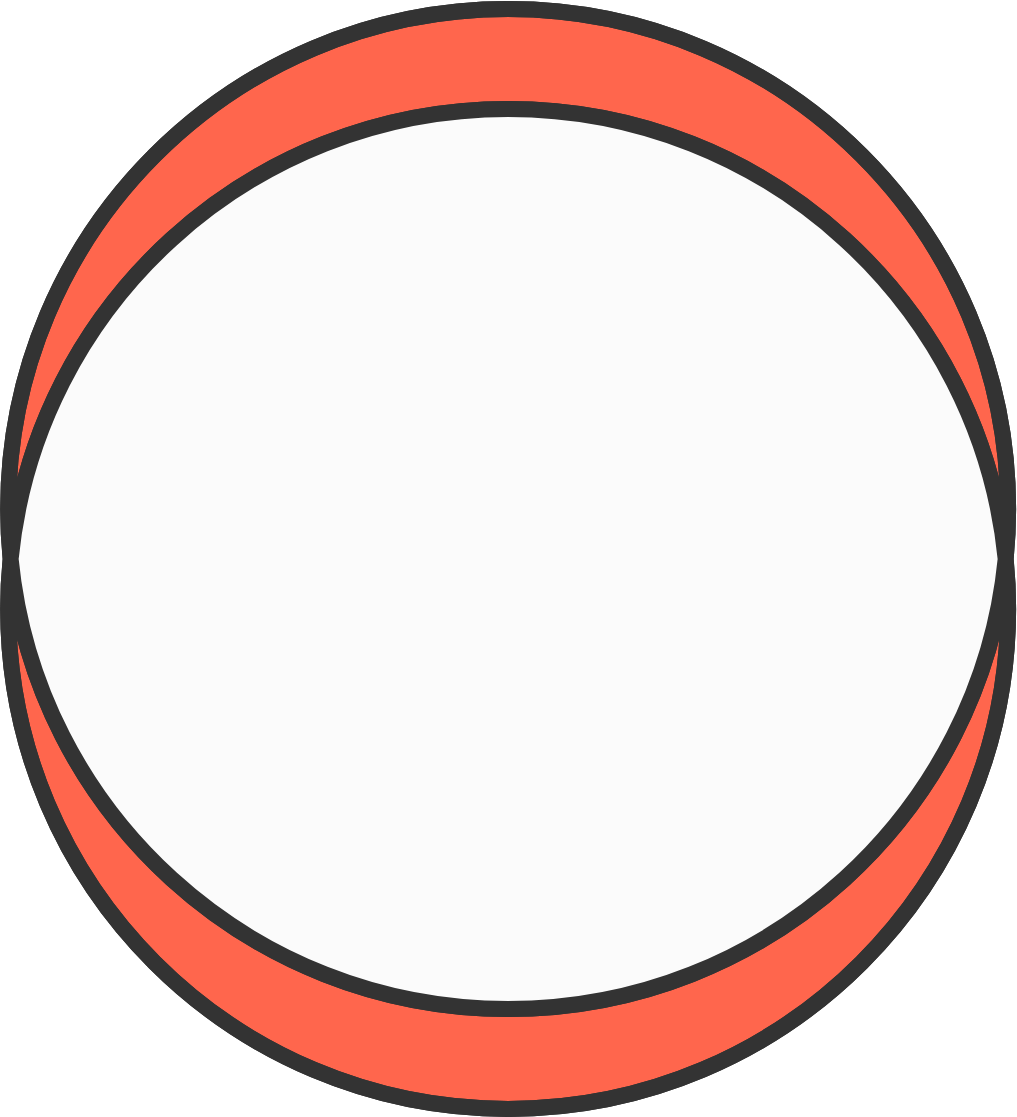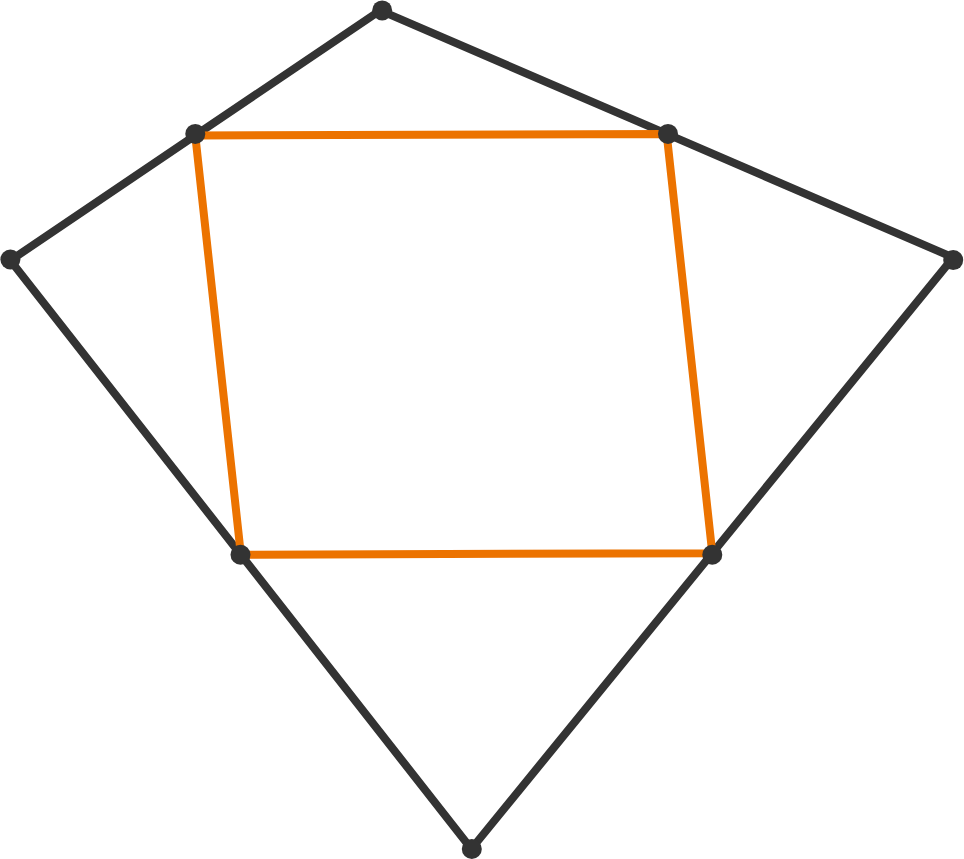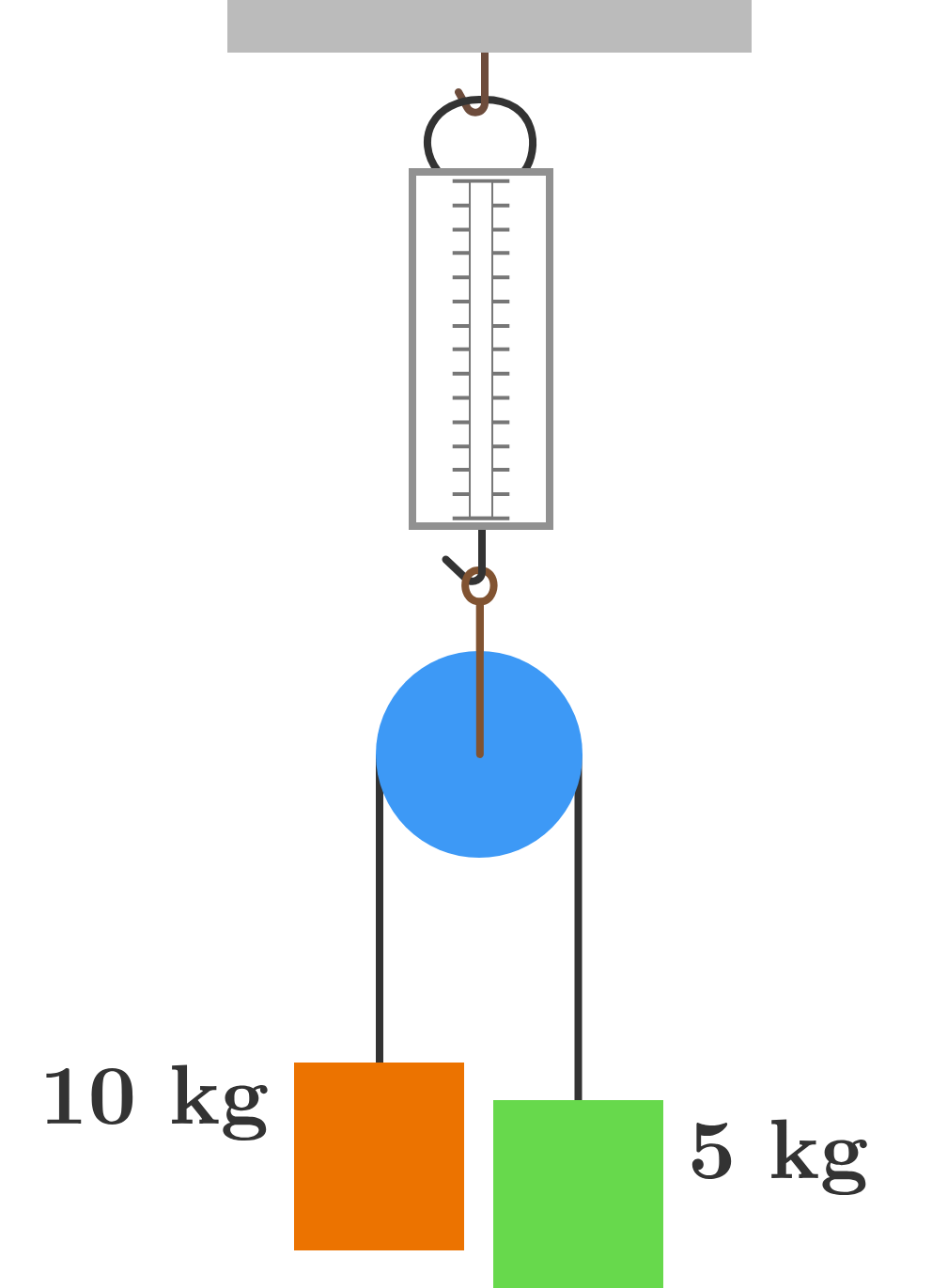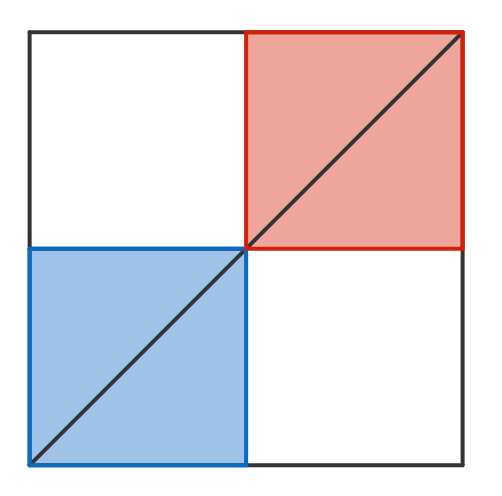# Problems of the Week

Contribute a problem

# 2018-09-24 IntermediateTwo congruent circles of radius $\text{5 cm}$ are drawn so that their centers are $\text{1 cm}$ apart.

What is the combined area of the two red-colored regions to the nearest square centimeter?

The midpoints of a quadrilateral are joined to form a parallelogram.

If the area of the parallelogram is half the area of the quadrilateral, what could the shape of the quadrilateral be?Select one or moreManya hung two blocks of respective masses $10 \text{ kg}$ and $5 \text{ kg}.$

What is the reading on the spring balance $($in $\text{kg})?$

Assumptions: The pulley and string are both massless and frictionless. The spring balance is calibrated to read the mass of a stationary object hung in the current gravity.Nine consecutive integers are placed in the squares of a $3 \times 3$ grid.

Every column, every row, and every diagonal add up to the same number $n$ and the middle number is $8.$

What is $n?$There are $2$ squares of side length $1$ that begin in opposite corners of a larger $2 \times 2$ square. Both squares slide diagonally towards each other (at the same speed) until they are in the corners opposite to those they started in. This process is then repeated in the opposite direction.

What is the average area of overlap between the two squares?

×